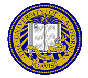# Homework 3

Due: November 8, 2021
Points: 100

In the given examples, what you type is in red and the program prints what is in black. Your program output should look exactly like the output in the examples, except that what you type won’t be in red.

1. (50 points) The birthday problem asks how many people must be in a room so that the probability of two of them having the same birthday is 0.5. This problem has you explore it by simulation. Basically, you will create a series of lists of random numbers of length n = 2, …, and look for duplicates. You will do this 5000 times for each length. For each length, count the number of lists with at least 1 duplicate number; then divide that number by 5000. That is the (simulated) probability that a list of n generated numbers has at least one duplicate. As the random numbers you generate are between 1 and 365 (each one corresponding to a day of the year), this simulates the birthday problem.

Now, breathe deeply and calm down. We will do this in steps!

1. First, detecting duplicates. Write a function called hasduplicates(l) that takes a list l and returns True if it contains a duplicate element, and False if it does not. For example:

```
>>> hasduplicates([1, 2, 3, 4, 5, 5, 2])↵
True
>>> hasduplicates([1, 2, 3, 4, 5, 6, 7])↵
False
```

2. Now, deal with one set of birthdays. Write a function called onetest(count) that generates a list of count random integers between 1 and 365 inclusive, and returns True if it contains a duplicate element, and False if it does not. Please use the function hasduplicates(l) to test for duplicates.

Hint: To generate a random number between a and b inclusive, put

```
import random
```
at the top of the program, and then call the function random.randint(a, b).

3. Now for the probability for count people. Write a function probab(count, num) that runs num tests of count people, and counts the number of tests with duplicates. It returns the fraction of the tests with duplicates; that is, the number of duplicates divided by num.

4. Now for the demonstration. Start with 2 people, and begin adding people until the probability of that many people having two people with a birthday in common is over 0.5. (In other words, start with a list of 2 elements, and increase the number of elements in the list until the simulation shows a probability of 0.5 that a number in the list is duplicated.) Print each probability; your output should look like the following:

```
For  2 people, the probability of 2 birthdays is 0.00220
For  3 people, the probability of 2 birthdays is 0.00880
For  4 people, the probability of 2 birthdays is 0.01680
For  5 people, the probability of 2 birthdays is 0.02940
For  6 people, the probability of 2 birthdays is 0.03940
For  7 people, the probability of 2 birthdays is 0.05900
For  8 people, the probability of 2 birthdays is 0.06840
For  9 people, the probability of 2 birthdays is 0.09700
For 10 people, the probability of 2 birthdays is 0.12360
and so forth
```

Also print the number of people needed so that the probability of two of them with a birthday in common is over 0.5 after the list of probabilities, like this:

```
You need 12 people for two of them to have the same birthday with probability at least 0.50000
```

Of course, the number 12 is highly improbable!

Hint: Don’t be surprised if your probabilities are slightly different than the ones shown in the sample output. As randomness is involved, it is very unlikely your numbers will match the ones shown here.

2. (50 points) The file data_hw_3.txt contains lines with 3 columns separated by a space representing the volume of air inspired and expired. The first column is the breath number (BN); the second column is the volume of air inspired, in ml; and the third column of air is the volume of air expired.

Write a program that computes the average, standard deviation, maximum difference between air inspired and expired for each breath, and the minimum difference between air inspired and air expired for each breath. Your output should look like this (but with the correct numbers; the ones here are wrong):

```
Average volume of air inspired is 120.15 ml with a standard deviation of 328.90
Average volume of air expired is 121.82 ml with a standard deviation of 561.20
The maximum difference between air inspired and expired is 289
The minimum difference between air inspired and expired is 5
```

### Extra Credit

1. (30 points) Define the function:The Collatz conjecture says that, if you iterate this sequence for any initial value of n, then eventually the sequence will reach the number 1.

For a given number n, let k be the least number of iterations needed to reach the number 1 (excluding the initial value). Then k is called the total stopping time of n.

For example, if n = 29, then the sequence is:

```
29 88 44 22 11 34 17 52 26 13 40 20 10 5 16 8 4 2 1
```
and so the total stopping time of 29 is 18.

Write a program that takes as input a positive integer and prints both the sequence and the total stopping time for that integer. The output should look like:

```
29 88 44 22 11 34 17 52 26 13 40 20 10 5 16 8 4 2 1
The total stopping time for 29 is 18
```Matt Bishop Office: 2209 Watershed Sciences Phone: +1 (530) 752-8060 Email: mabishop@ucdavis.edu